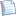# Review P: C Pointersprintable version

P1:       // P2:        // P3:            

### Problem P1.1

What does each of the following C programs display?

 a. ```#include  int main() {     int i;  int j;     int *p; int *q;     p = &i;     q = &j;     i =  9;     j =  8;     p =  q;     *q = 7;     printf("%d %d\n", i, j);     printf("%d %d\n", *p, *q);     return 0; } ``` b. ```#include  int main() {     int i;  int j;     int *p; int *q;     p = &i;     q = &j;     i =  3;     j =  5;     *q = 8;     q =  p;     printf("%d %d\n", i, j);     printf("%d %d\n", *p, *q);     return 0; } ```